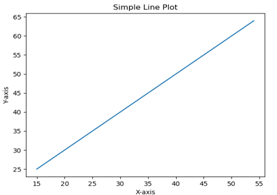# What is Matplotlib in Data Visualization? | Python crash course_03

## What is Matplotlib in Data Visualization?

### Hello everyone, welcome to Python crash course. Today I am going to Explain What is Matplotlib in Data Visualization? Let's Start:

A pictures is worth a thousand word, and with Python’s matplotlib library, it, fortunately, take far less than a thousand words of code to create a production-quality graphic.However, matplotlibs is also a massive library, and getting a plot to looks just right is often achieved through trial and error. Using one-liners to generate basic plot in matplotlib is fairly simple, but skillfully commanding the remaining 97% of the library can be daunting.
This articles is a beginner-to-intermediate-level walkthrough on matplotlib that mixe theory with example. While learning by example can be tremendously insightful, it helps to have even just a surface-level understanding of the library’s inner working and layout as well.
SOME EXAMPLE OF MATPLOTLIB:
In this chapter, we will learn how to create a simple plots with Matplotlib.
We shall now display a simple line plots of angle in radians. its sine values in Matplotlib. To begin with, the Pyplot module from Matplotlib package is imported, with an alias plot as a matter of convention.
`import matplotlib.pyplot as plt`
Next we need an array of number to plot. Various array function are defined in the Numpy library which is imported with the np alias.
`import numpy as np`
We now obtain the ndarray object of angle between 0 and 2π using the arange() function from the Numpy library.
`x = np.arange(0, math.pi*2, 0.05)`
The ndarray object serves as values on x axis of the graphs. The corresponding sine values of angles in x to be displayed on y axis are obtained by the following statements −
`y = np.sin(x)`
The value from two arrays are plotted using the plot() functions.
`plt.plot(x,y)`
You can set the plots title, and labels for x and y axes.
```
#You can set the plots title, and labels for x and y axes.

plt.xlabel("angle")

plt.ylabel("sine")

plt.title('sine wave')
```

The Plots viewer window is invoked by the show() function −
`plt.show()`
The complete programs is as follow −
```
from matplotlib import pyplot as plt

import numpy as np

import math #needed for definition of pi

x = np.arange(0, math.pi*2, 0.05)

y = np.sin(x)

plt.plot(x,y)

plt.xlabel("angle")

plt.ylabel("sine")

plt.title('sine wave')

plt.show()
```